# Frequency Divider Circuit using 555 Timer and CD4017

Published  January 17, 2018   1
S
Author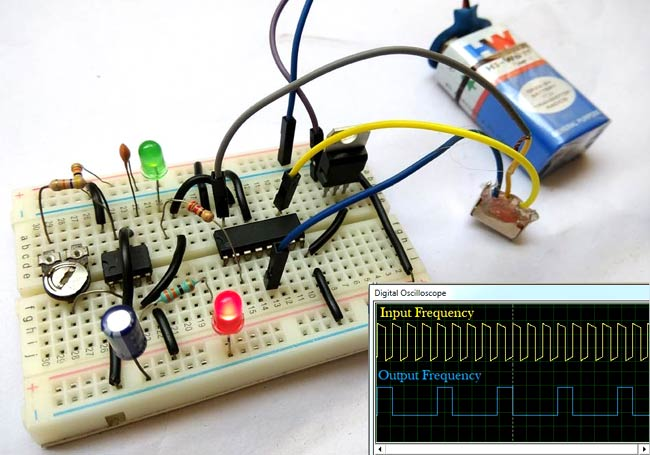Frequency Dividers are the circuits which divide the input frequency by n (any integer number), means if we provide some signal of frequency ‘f’ then the output will be the divided frequency ‘f/n’. Frequency dividers are very useful in analog as well as digital applications. Here we are building the circuit to divide the frequency by 2 or 4.

In this circuit, we have used Astable multivibrator by using 555 timer IC to generate input signal of frequency ‘f’. Now, in the second phase, we have used a decade counter IC 4017 to divide this input signal frequency by f/2 or f/4. Input frequency can be adjusted by using RV1 potentiometer and output frequency can be switched between f/2 and f/4 by using the SPDT switch.

### Components Required:

1. 555 Timer IC
2. 4017 counter IC
4. Resistor 330, 220, 10K, 47k ohm
5. 50k POT
6. LEDs
7. 4.7uF Capacitor
8. 10nF Capacitor
9. SPDT switch
10. Jumper wire
11. 9V battery or supply
12.  Voltage Regulator LM7805

### Circuit Diagram and Explanation: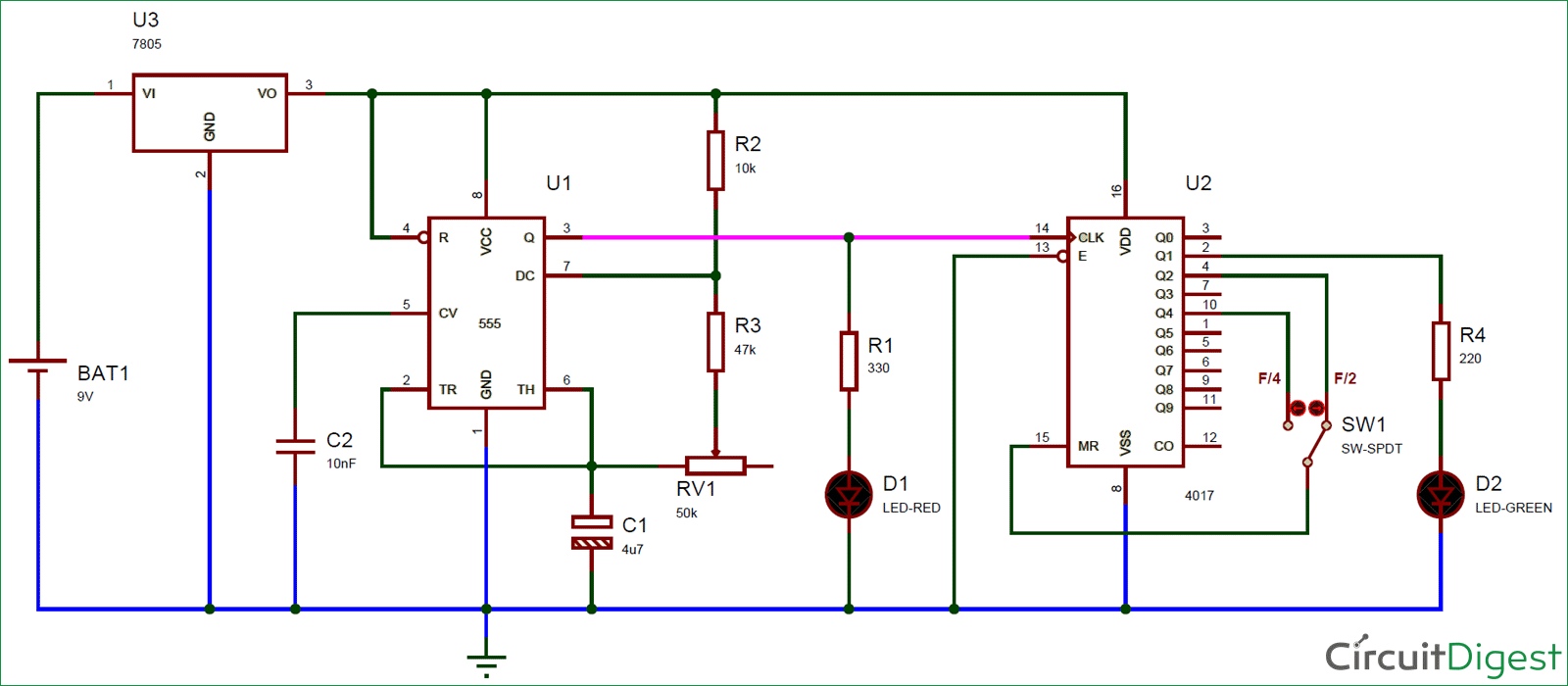In this Frequency Divider Circuit, we have used a 555 timer IC to generate an input frequency signal. Here we have connected a 10k (R2) resistor between Vcc and pin 7th of 555 Timer (U1). Then we have connected 47k (R3) resistor & 50k Pot (RV1) between pin 7 and 6. Pin 2 is shorted with pin 6 and a 4.7uF capacitor C1 is connected to pin 2 or 6 with respect to ground. Pin 1 is connected to ground and pin 4 directly connected to VCC and pin 8 as well. The output pin of this 555 timer is connected to a LED D1 through a 330-ohm resistor and also connected to clock pin of 4017 counter IC. LED D1 will indicate the frequency of input signal.

4017 Counter IC is responsible for dividing the frequency by f/2 or f/4. An SPDT switch is used for selecting frequency. A LED D2 is connected to pin 2 of IC 4017 through a 220-ohm resistor, which indicates the divided frequency. Means LED D1 will blink with frequency f and LED D2 will blink with frequency f/2 or f/4 depending upon the position of SPDT switch. A 7805 IC is used to regulate the voltage. Finally, we have connected a 9v Battery to power the circuit.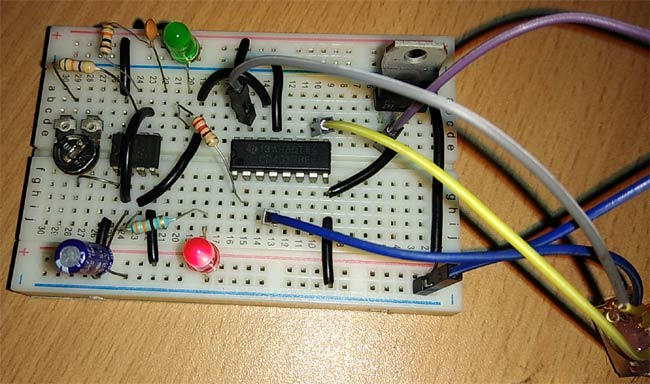Before going further we should understand the working of 4017 IC.

### Working Explanation:

Working of this Frequency Divider Circuit is simple. Here we have made a 555 based astable multivibrator for the input signal and we are controlling the frequency of the signal by using a potentiometer.

When we connect supply to circuit then Astable Multivibrator generates a frequency which can be easily seen by the blinking LED D1. This signal is applied to the clock input of counter IC 4017 as a clock pulse.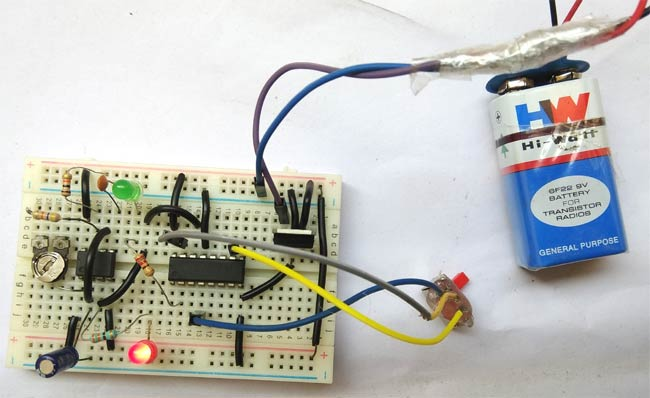In case of the frequency divided by 2 (f/2), we have applied the Q2 output to reset pin (15) of counter IC by using SPDT switch so that the counter IC reset itself and starts from the beginning (Q0). Means for first clock pulse output Q1 will be high and for second clock pulse output Q2 will be high which resets the IC and makes the output Q0 high. For third clock pulse output Q1 will be high again and LED will glow. So for every two input clock pulse, LED D2 will be high once, that how it divides the frequency by 2. So the final output of the counter IC will be: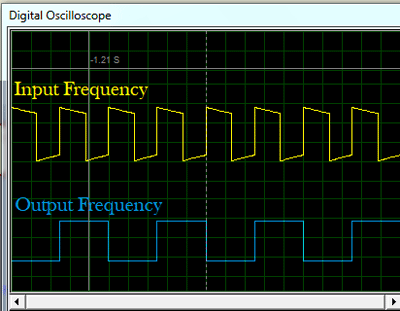In case of frequency divided by 4 (f/4), we have applied the Q4 output to reset pin (15) of counter IC by using SPDT switch so IC 4017 will be reset in the fourth pulse, hence LED D2 will glow once during four pulses. Initially, Q0 will be high that is the default state of IC, then for first clock pulse output Q1 will be high and LED D2 will glow. For second and third clock pulses, output Q2 and Q3 will be high respectively. Now in fourth pulse Q4 gets high and resets the IC as it is connected to reset Pin 15 of IC 4017 (Q0 high). For fifth clock pulse output Q1 will be high again and LED will glow. So here, for every four input clock pulses, LED D2 will be high once, that how it divides the frequency by 4 (f/4).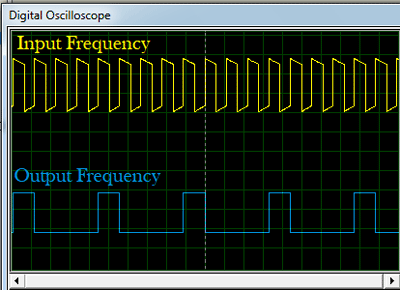A video for the complete working of Frequency Divider Circuit is given below.

Video